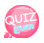# do ~ while loop statement

It is similar to the while statement. The difference is that do is attached.
The do-while statement executes the first time even if do does not meet the conditions.
The order of the syntax differs from the while statement.
Write the statement in the do statement as shown in the source below. Then
After it is executed once, the while statement written after it is activated, it distinguishes between true and false and if it is true, executes the execution statement written in the do statement. That is, run the do statement above rather than below.

```\$num = 1;
do{
echo " {\$num} ........ {\$a} <br  />";
\$a++;
}
while(\$a<=10);
```

Then figure out do while again through the source!

```<?php
echo "Sum 1 to 10 using do ~ while statement <hr />";

\$a = 1;        // Initial value declaration
\$sum = 0;      // Cumulative Sum Variable Declaration

do {
echo "Cumulative sum up to ".\$a."(the value of \\$num) ";
\$sum +=\$a;
\$a++;
echo "={\$sum} <br />";
}
while(\$a <= 10);

echo "Sum from 1 to 10{\$sum} <br />";
?>```declare a as 1 Declare the variable sum to store the cumulative sum.
do-while statement
Run it once.
Then a is 1, so the cumulative sum up to 1(the value of \$sum) = 1
Is expressed. By the increment, a is assigned 2, so the do statement ends
Execution is executed until a is 10 in the while statement.